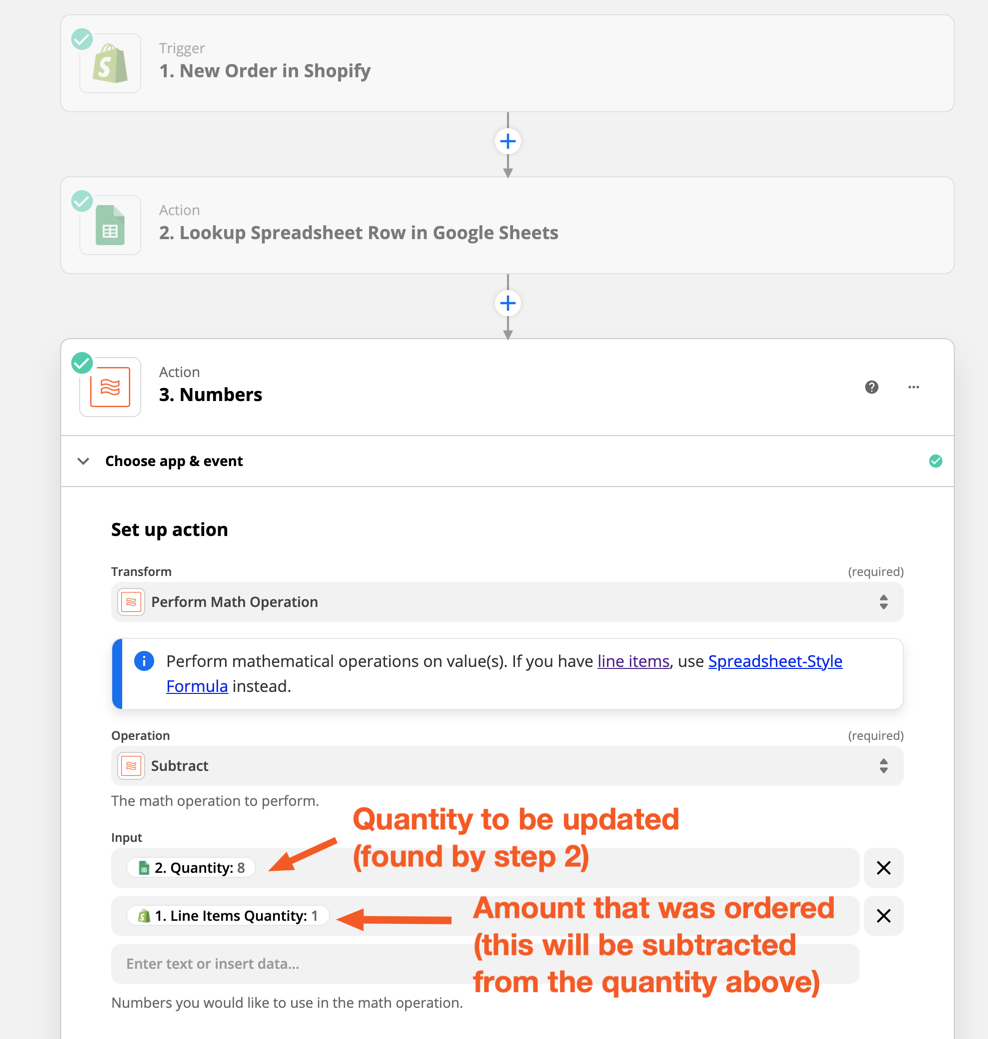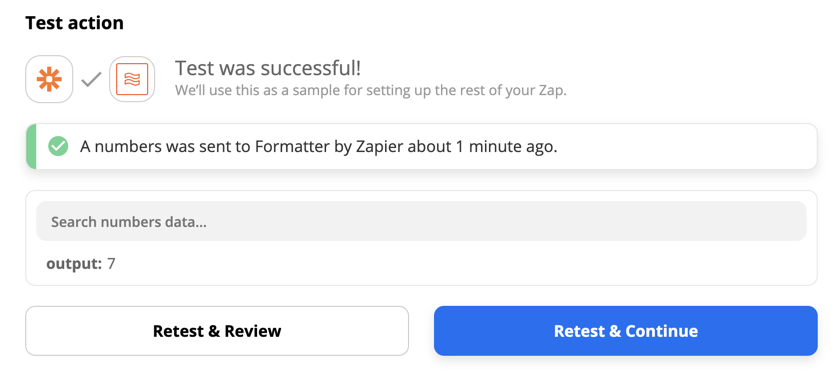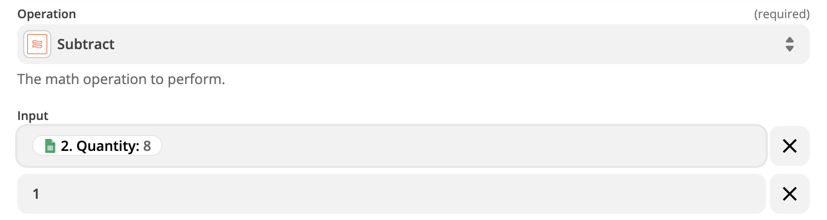# How to reduce a number value by 1 in Google Sheets row

• 2 replies
• 39 views

• New
• 0 replies

So let’s say I’m counting inventory in a Google Spreadsheet and every time someone sends a payment I want the stock number to be reduced by 1.

I currently use Zapier’s ‘Update Spreadsheet Row’ action, but the problem is that I can only replace the content of a cell, but I can’t just reduce the value by the amount of 1.

So let’s say the value of that cell is 100 and I want to deduct 1 every time an action is triggered. How do I do that?

icon

Best answer by SamB 1 September 2021, 17:58

View original

### 2 replies

Userlevel 7+11
• Troy Tessalone• Moderator & Certified Expert
• 5173 replies

Hi @K_B

Try this…

Action: GSheets - Lookup/Find Row

Action: Formatter > Number > Perform Math Operation (Subtract 1)

Action: GSheets - Update Row

Userlevel 6+8
• SamB• Community Manager
• 760 replies

Just wanted to follow up here to expand on Troy’s suggestion to show how to set up the Formatter step for cases where more than 1 of the same item is ordered.

In the example below I’ve step up a Zap that uses the Shopify New Order trigger, but the process should work for any other app that’s sending the quantity ordered value to the Zap.

• After the trigger step, a Lookup Spreadsheet Row action (step 2), would be used to search the spreadsheet for the relevant item that was ordered. More details on how to set up search actions like the Lookup Spreadsheet Row one can be found here: Search for existing data in Zaps
• Then in a Formatter (Numbers > Perform Math Operation - Subtract) step, you’d select the column that contains the quantity for the relevant item in the Spreadsheet. And select the amount that was ordered from the trigger step:So when we test that action step, the amount ordered (1) is subtracted from the quantity (8), giving us the correct amount of 7:You would then select this output to use as the quantity value in the Update Spreadsheet Row step. This would mean that if 3 of the same item were ordered in a single order, the quantity in the spreadsheet would be reduced by 3.

That being said, if it was only possible for one item to be purchased in an order then, instead of selecting the amount that was ordered you could just type in 1. This would mean that every time an order was placed the quantity found by step 2 would have 1 subtracted each time. For example:You can find out more about using Formatter (Numbers > Perform Math Operation) actions here: